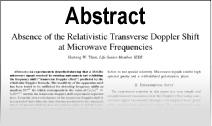Enter the content which will be displayed in sticky bar

AbstractOn the General Solution to Einstein's Vacuum Field for the Point-Mass when lambda ???0 and Its Consequences for Relativistic Cosmology

Year: 2005
Keywords: Einstein's Vacuum Field, Point-Mass, Relativistic Cosmology
It is generally alleged that Einstein?fs theory leads to a finite but unbounded universe. This allegation stems from an incorrect analysis of the metric for the point-mass when ?? <> 0. The standard analysis has incorrectly assumed that the variable r denotes a radius in the gravitational field. Since r is in fact nothing more than a real-valued parameter for the actual radial quantities in the gravitational field, the standard interpretation is erroneous. Moreover, the true radial quantities lead inescapably to ??=0 so that, cosmologically, Einstein?fs theory predicts an infinite, static, empty universe